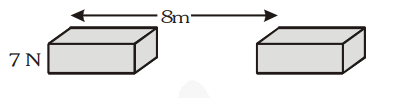# A force of 7 N acts on an object. The displacement is,

Question.
A force of 7 N acts on an object. The displacement is, say 8 m, in the direction of the force (see fig.). Let us take it that the force acts on the object through the displacement. What is the work done in this case ?Solution:

Given, force = 7 N ; displacement = 8 m

Work done = Force × Displacement

$=7 \times 8=56 \mathbf{J}$